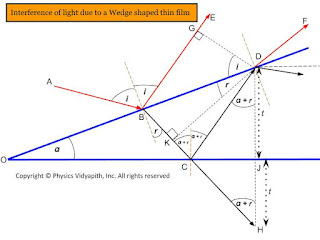### Interference of light due to a wedge shaped thin film

Derivation of interference of light due to a wedge-shaped thin film:Interference of light due to wedge-shaped thin film

The wedge-shaped film is bound by two plane surfaces inclined at angle $\alpha$.

The thickness t of the film varies uniformly from zero at the edge to its maximum value at the other end.

A Ray of light ab incident on the film will be partially reflected along be and partially transmitted along $BC$. The ray $BC$ will be partially reflected along $CD$ which will be again partially transmitted along $BF$. The two rays $BE$ and $DF$ in reflected light diverge.

The path difference between ray $BE$ and $DF$ is

$\Delta=\mu(BC+CD)-BG \qquad(1)$

Where $\mu$- Refractive index of the film

$\Delta CDJ$ and $\Delta CHJ$ are congruent so

$\begin{Bmatrix} CD=CH \\ DJ=JH=t \end{Bmatrix} \qquad(2)$

From equation $(1)$

$\Delta=\mu(BK+KC+CH)-BG \qquad (\because BD=BK+KC)$

$\Delta=\mu(BK+KH)-BG \qquad (3)$

In $\Delta BDG$-

$sin\:i = \frac{BG}{BD}$

$BG=BD sin \: i \qquad(4)$

In $\Delta BDK$

sin\:r =\frac{BK}{BD}

$BD=\frac{BK}{sin \: r} \qquad(5)$

From equation $(4)$ and equation $(5)$-

$BG=BK. \frac{sin \: i}{sin \: r}$

$BG=\mu . BK \qquad(6) \qquad (\because \mu=\frac{sin \: i}{sin \: r})$

Now put the value of $BG$ in equation $(3)$

$\Delta= \mu(BK+KH)- \mu. BK$

$\Delta= \mu.KH \qquad(7)$

In $\Delta DKH$

$cos \: (\alpha + r)= \frac{KH}{DH}$

$KH=DH \: cos \: (\alpha + r)$

$KH=(DJ+JH) \: cos \: (\alpha + r)$

$KH=2t \: cos \: (\alpha + r) \qquad(8)$ {From equation $(2)$}

Now put the value of $KH$ in equation $(7)$

 $\Delta= 2\mu t \: cos \: (\alpha + r)$

The ray $DF$ suffers a phase change of $\pi$ due to reflection from a denser medium at $C$. Whereas there is not any change in phase due to reflection for ray $BE$.

 $\Delta= 2\mu t \: cos \: (\alpha + r) \pm \frac{\lambda}{2}$

For Constructive Interference:

$\Delta= n \lambda$

$\Delta= 2\mu t \: cos \: (\alpha + r) \pm \frac{\lambda}{2}= n \lambda$

 $2\mu t \: cos \: (\alpha + r)= (2n \pm 1) \frac{\lambda}{2}$

For Destructive Interference:

$\Delta= (2n \pm 1) \frac{\lambda}{2}$

$\Delta= 2\mu t \: cos \: (\alpha + r) \pm \frac{\lambda}{2}=(2n \pm 1) \frac{\lambda}{2}$

 $\Delta= 2\mu t \: cos \: (\alpha + r) =2n \lambda$